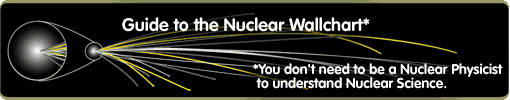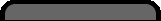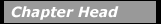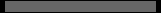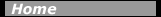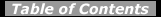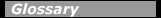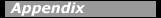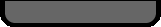## The Nucleus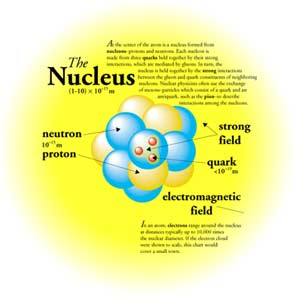The nucleus depicted above is now understood to be a quantum system composed of protons and neutrons, particles of nearly equal mass and the same intrinsic angular momentum (spin) of 1/2. The proton carries one unit of positive electric charge while the neutron has no electric charge. The term nucleon is used for either a proton or a neutron. The simplest nucleus is that of hydrogen, which is just a single proton, while the largest nucleus studied has nearly 300 nucleons. A nucleus is identified as in the example below by its atomic number Z (i.e., the number of protons), the neutron number, N, and the mass number, A, where A = Z + N.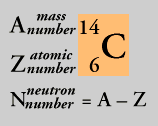The convention for designating nuclei is by atomic number, Z, and mass number, A, as well as its chemical symbol. The neutron number is given by N = A - Z.

What else do we know about the nucleus? In addition to its atomic number and mass number, a nucleus is also characterized by its size, shape, binding energy, angular momentum, and (if it is unstable) half-life. One of the best ways to determine the size of a nucleus is to scatter high-energy electrons from it. The angular distribution of the scattered electrons depends on the proton distribution. The proton distribution can be characterized by an average radius. It is found that nuclear radii range from 1-10 ´ 10-15 m. This radius is much smaller than that of the atom, which is typically 10-10 m. Thus, the nucleus occupies an extremely small volume inside the atom. The nuclei of some atoms are spherical, while others are stretched or flattened into deformed shapes.

The binding energy of a nucleus is the energy holding a nucleus together. This energy varies from nucleus to nucleus and increases as A increases. Because of variations in binding energy, some nuclei are unstable and decay into other ones. The rate of decay is related to the mean lifetime of the decaying nucleus. The time required for half of a population of unstable nuclei to decay is called the half-life. Half-lives vary from tiny fractions of a second to billions of years.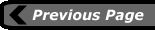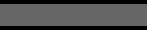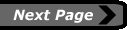last updated: August 9, 2000 webmaster